Electricity - Circuits and graphs
10 MCQs questions on electricity
Email address *
Your Name *
Your answer
Class/section *
Your answer
Name of your school *
Your answer
1. In an electric circuit three incandescent bulbs A, B and C of rating 40W, 60W and 100W respectively are connected in parallel to an electric source. Which one of the following is likely to happen regarding their brightness? *
1 point
2. Which one of the following statement is correct? *
1 point
Study the diagram and answer Q3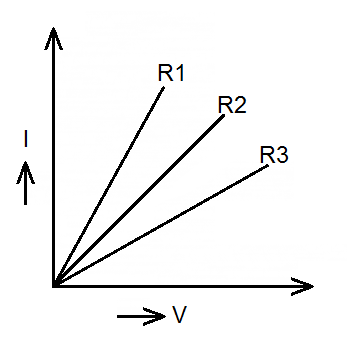3. A student carries out an experiment and plots the V-I grpah of three samples of nichrome wire with the resistances R1, R2 and R3 respectively. Which of the following is true? *
1 point
Study the diagram and answer Q4.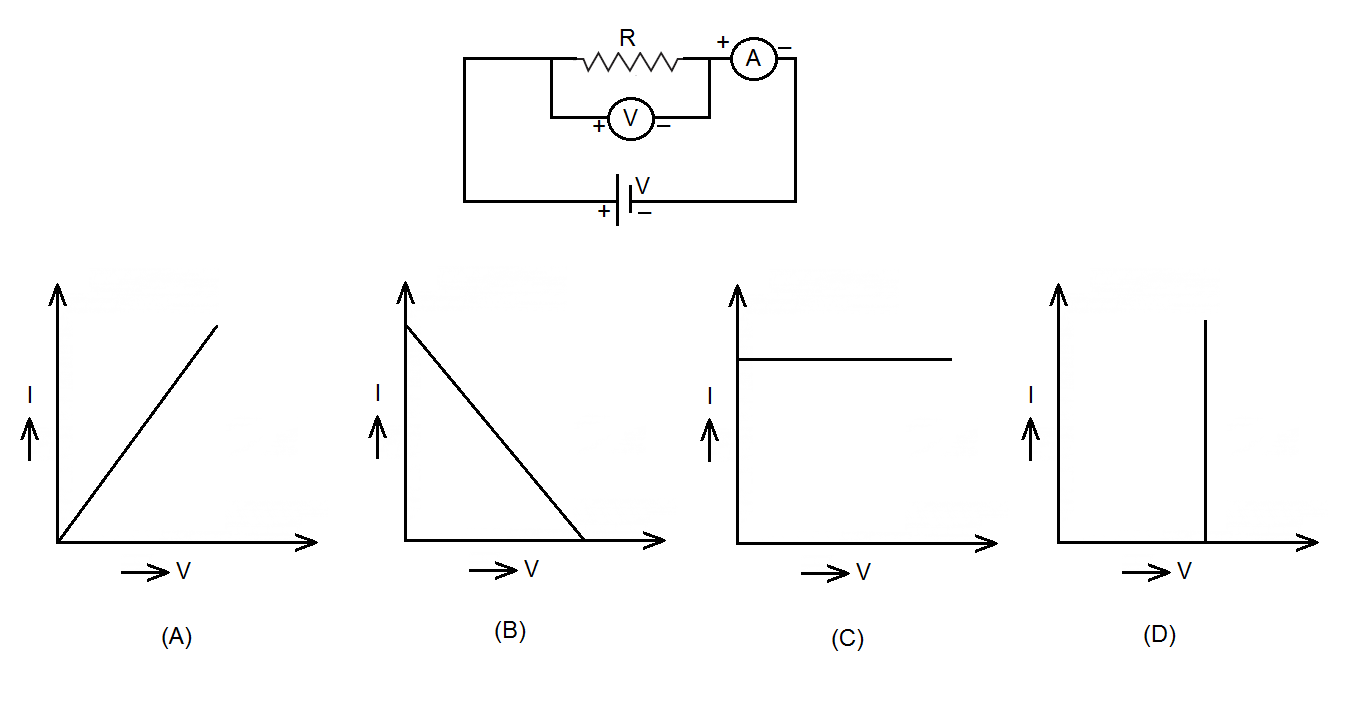4. Using the circuit given above, current and potential difference were measured and these values were plotted on a graph. Which one of the following graphs most correctly shows the dependence between current and potential difference? *
1 point
5. In a voltmeter, there are 20 divisions between the 0 mark and 0.5 V mark. The least count of the voltmeter is *
1 point
Study the diagram and answer Q6.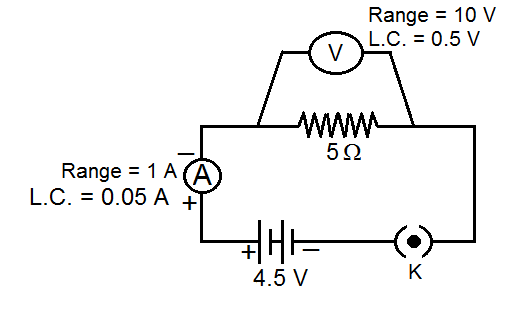6. The voltmeter, ammeter and resistance in the circuit shown have been checked to be correct. On plugging the key, the ammeter reads 0.9 A, but the voltmeter reads zero. This could be because *
1 point
Study the diagram and answer Q7.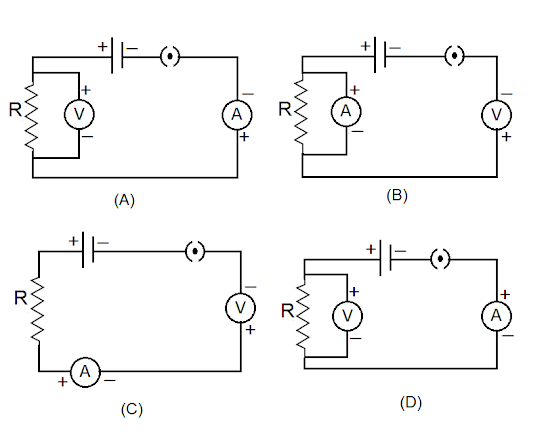7. Identify the circuit in which the electrical components have properly connected. *
1 point
Study the diagram and answer Q8.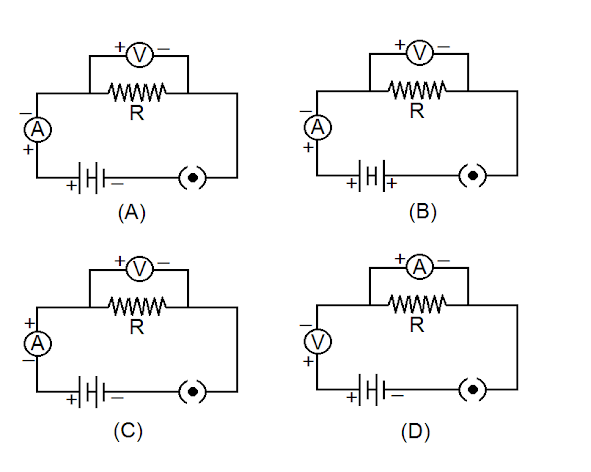8. Four students A, B, C and D set up their circuits for studying the dependence of the current through a resistor on the potential difference across it, as follows. The correct circuit is *
1 point
Study the diagram and answer Q9.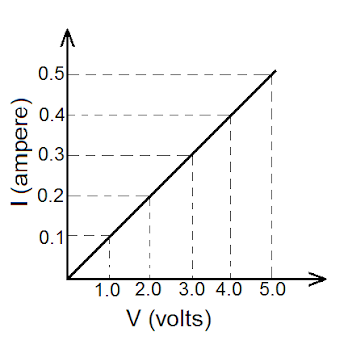9. In an experiment to study the dependence of current on potential difference across a resistor, a student obtained the graph as shown in the diagram. The value of resistance of the resistor is *
1 point
Study the diagram and answer Q10.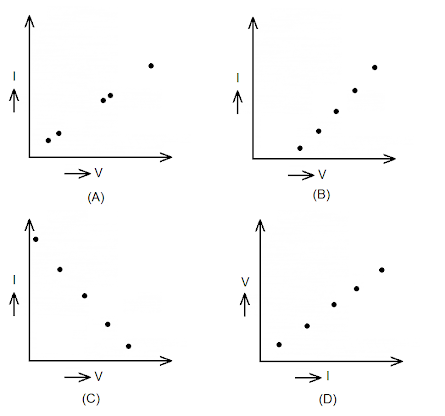10. The plot correctly showing the dependence of the current I on the potential difference V across a resistor R is *
1 point
Never submit passwords through Google Forms.
This content is neither created nor endorsed by Google. Report Abuse - Terms of Service